Get instant live expert help with Excel or Google Sheets“My Excelchat expert helped me in less than 20 minutes, saving me what would have been 5 hours of work!”

#### Post your problem and you’ll get expert help in seconds.

Your message must be at least 40 characters
Our professional experts are available now. Your privacy is guaranteed.

# How to Find Standard Deviation in Google Sheets and Excel – Excelchat

Excel enables us to calculate the standard deviation of any data set in no time by using one of these functions:  STDEV, STDEVP, STDEV.S, STDEVA. STDEV.P, STDEVPA.

Now that’s just confusing.

But with the following checklist, we will be able to determine the appropriate function to use:

• What Excel version are we using?  Are we still using version 2007 and earlier?
• Do the arguments represent just a sample of the population or the entire population?
• Do we ignore the text and logical values, or evaluate them?
• Note: Text and the logical “FALSE” are evaluated as “0” while “TRUE” is evaluated as “1”

We answer those three questions and refer to the table below: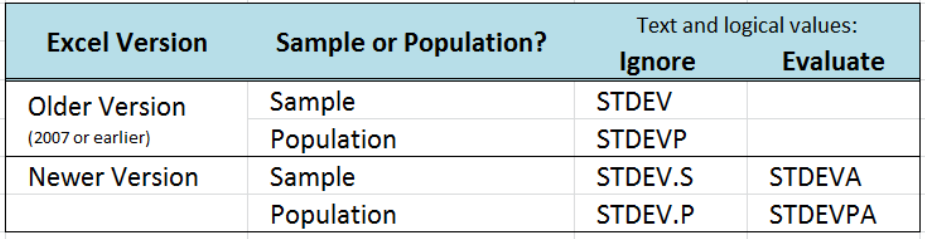Figure 1.  Table guide for which standard deviation function to use

This table will help us understand the different uses and relevance of the standard deviation functions.  It guides us through which function to use in Excel for standard deviation if we consider the entire population or just a sample size.

## Sample problem 1

Which function in Excel do we use to calculate the standard deviation of a sampled data set?

To calculate standard deviation in Excel with a sampled data set, we can use the STDEV, STDEV.S or STDEVA functions.  In order to determine which function to use, let us walk through the checklist:

• What Excel version are we using?  Are we still using version 2007 and earlier?

• Do the arguments represent just a sample of the population or the entire population?

Sample

• Do we ignore the text and logical values, or evaluate them?

Ignore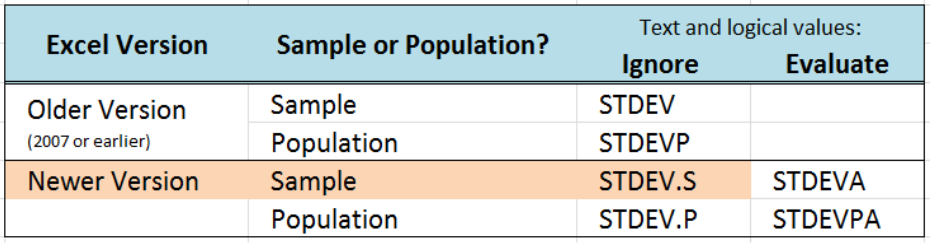Figure 2.  Table guide showing STDEV.S as the best function to use

Referring to our checklist and table, if we are using a newer version of Excel and we want to ignore the text and logical values, the function to use is STDEV.S.

## Sample problem 2

Excel standard deviation p versus s

As discussed above, the checklist and the table will guide us in deciding whether to use the Excel STDEV.P or STDEV.S when we calculate for standard deviation. It is critical to use the correct function in order to obtain accurate results.

### Example

There are 10 students in a class and their scores in a test are given in the table below.  Compute for the standard deviation.

### Solution

Since there are only 10 students in the class, the 10 scores represent the entire population of data.  Using the newer version of Excel and ignoring text and logical values, the table shows that we must use STDEV.P to calculate the standard deviation.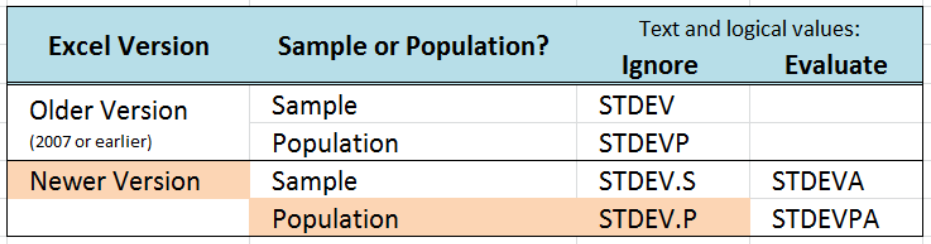Figure 3.  Table guide showing STDEV.P as the best function to use

### Syntax of STDEV.P

`=STDEV.P(number1,[number2],...])`

• number1   – any value, cell reference or range corresponding to a population, for which we want to compute for standard deviation
• only number1 is required, succeeding numbers are optional

We enter the following formula in cell D3:

`=STDEV.P(B3:B12)`

As a result, the standard deviation of the scores is 6.4195.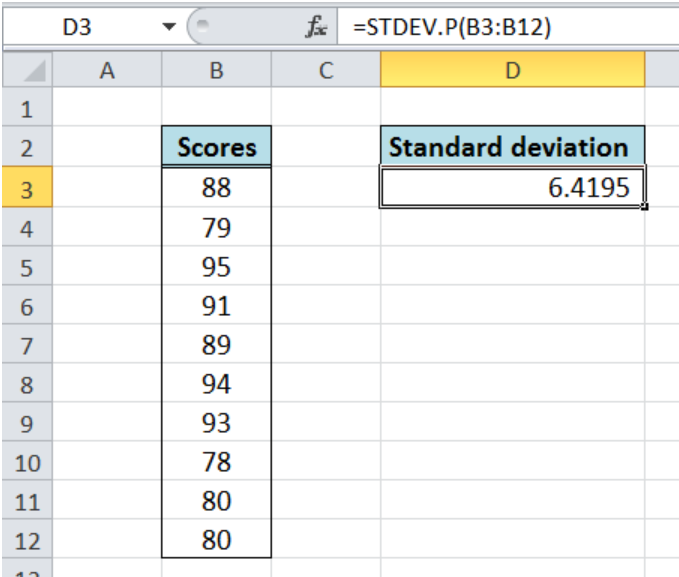Figure 4.  Entering STDEV.P formula to calculate standard deviation

Suppose we have used the function STDEV.S instead.  The result would have been different as shown below: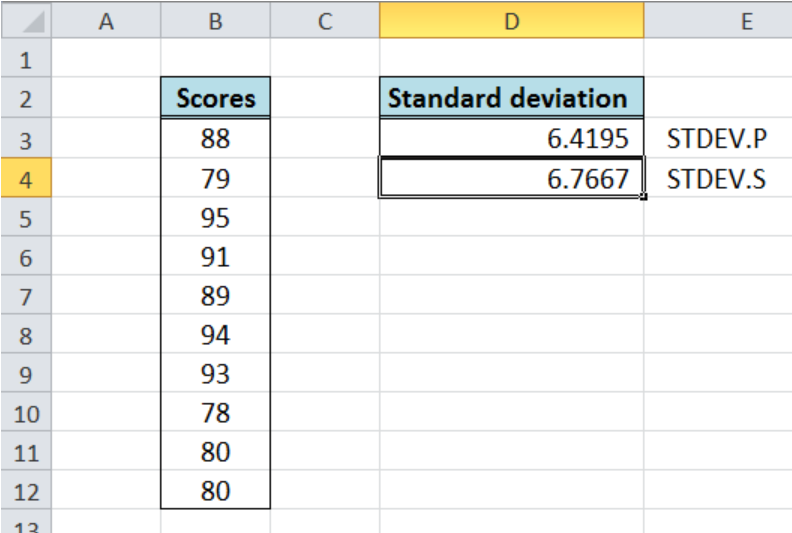Figure 5. Output: Excel standard deviation p versus s

Using the wrong function will lead to erroneous results.

## Sample problem 3

How to calculate Excel standard deviation with IF?

While working with Excel, we can categorize our data and calculate the standard deviation by incorporating the IF function.

Suppose we have a table showing ten scores of students with different levels.  We want to find the standard deviation per level. We follow these steps:

• Enter this formula in cell F3:  `=STDEV.S(IF(\$B\$3:\$B\$12=E3,\$C\$3:\$C\$12))`
• Copy the formula in cell F3 to cells F4:F5 by clicking the “+” icon at the bottom-right corner of cell F3 and dragging it down
• For each cell in F3:F5, we have to select the cell and press Ctrl + Shift + Enter to activate the array formula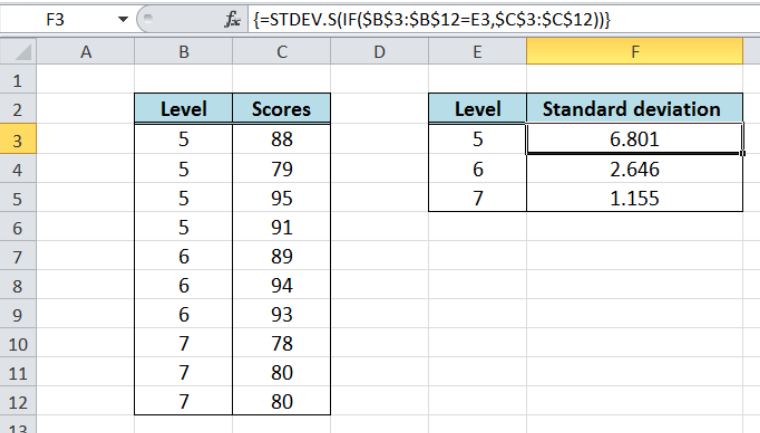Figure 6. Output: Excel standard deviation with IF

As a result, the standard deviation per level is calculated by using the STDEV.S function.

Most of the time, the problem you will need to solve will be more complex than a simple application of a formula or function. If you want to save hours of research and frustration, try our live Excelchat service! Our Excel Experts are available 24/7 to answer any Excel question you may have. We guarantee a connection within 30 seconds and a customized solution within 20 minutes.

Solution examplesCould you help me by explaining the IF function?
Solved by G. L. in 24 minsI have a question about Conditional Formatting. =IF('Sheet1'!A1="X",IF('Sheet1'!B1="Y", TRUE, FALSE),FALSE) the set the cell background to red. I would like to make it so that if Sheet1'!B1="Z" I set the back to blue. How can I achieve this.
Solved by B. F. in 39 minsI need to find out if the 2018 yearly sales goals were met if the yearly sales were \$25,000 or more using an IF logical function and to set the formula to return a value of YES if met, and NO if not
Solved by A. A. in 18 minsI filtered data in column A (it is labeled ID #) of a data set. On my next worksheet, column A is also the ID #, but it is a different data set. I want to filter the ID #'s the same for the two sheets, but how do I do that being that I am working with two different sets of data?
Solved by V. H. in 22 minsi want to create a very simple if function in a cell which is, if one cell is less than 1250, then the automatic value in the current cell is 250, dead easy, for someone who knows! Thank you.
Solved by A. H. in 14 mins